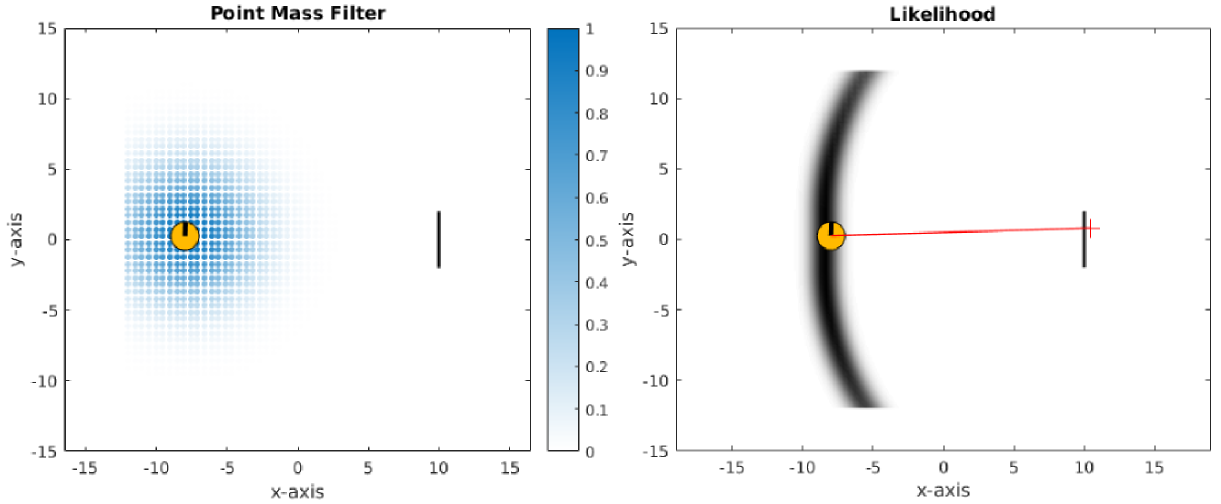# Point Mass FilterFigure: PMF example Left: Point Mass Filter: intensity of blue regions is proportional to the probability that the agent (orange pack-man) occupies this region. Right: Likelihood of the location the agent could be in given a measurement (range and bearing shown in red).

The Point Mass Filter (PMF) is the deterministic counter part of the Particle Filter (PF). That is the PMF does not require a re-sampling step. This is advantageous both computational speed and it considerably reduces the black art necessary for tuning the parameters of the PF.

Instead of representing the filtered probability distribution $$p(x_t|y_{0:t},u_{1:t})$$ by a set of samples drawn from a proposal distribution, as it is the case for PF, the state space $$x$$ is deterministically discretized and represented by a grid. The value of each grid point or cell contains a valid probability in contrast to the PF where a point or particle represents a sample drawn from a proposal distribution as the true probability distribution is unknown. The PMF directly estimates the filtered probability distribution.

Summary of the main differences (pros/cons) of the PMF and PF.

### PMF

• PMF grid values are probabilities whilst the PF particles are samples draw from an proposal distribution. If you wanted to get an estimation of the filtered probability when using a PF, you would have to extract it from the set of particles by either fitting a Gaussian Mixture Model to them (this is one example) or integrate the number of particles within discretized intervals.

• PMF has no re-sampling step which reduces computation and as a result there is no need to tune the hyper-parameters of a re-sampling kernel (usually a Gaussian) which is necessary for PF.

• PMF parameters are intuitive. You can set the minimum and maximum resolution of the grid which will dynamically resize as wanted.

• The PF is straight forward the implement, but hard to tune. The PMF is “harder” to implement but easy to tune.